# G.4 - 6 Which Trig Ratio?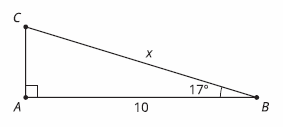Which ratio could be used to write an equation relating the sides and angle given in this picture?

Check all that apply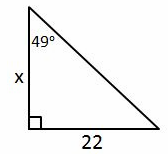Which ratio could be used to write an equation relating the sides and angle given in this picture?

Check all that apply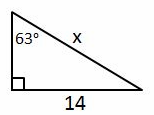Which ratio could be used to write an equation relating the sides and angle given in this picture?

Check all that apply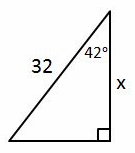Which ratio could be used to write an equation relating the sides and angle given in this picture?

Check all that apply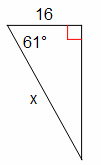Which ratio could be used to write an equation relating the sides and angle given in this picture?

Check all that apply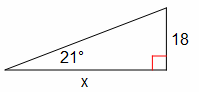Which ratio could be used to write an equation relating the sides and angle given in this picture?

Check all that apply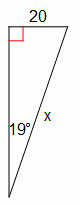Which ratio could be used to write an equation relating the sides and angle given in this picture?

Check all that apply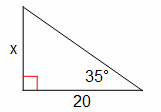Which ratio could be used to write an equation relating the sides and angle given in this picture?

Check all that apply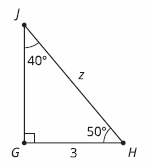Which two ratios could be used to write an equation relating 2 sides and an angle given in this picture?

Check all that apply